# Visualization: Mapping Global Earthquake Activity¶

This project introduces the Basemap library, which can be used to create maps and plot geographical datasets.

## Introduction¶

The main goal of this project is to help you get comfortable making maps of geographical data. If you follow along with the tutorial, you will end up making this map:It takes fewer than 50 lines of code to generate this map from a raw dataset! This project will conclude with a list of datasets to explore, to help you find a project of your own to try.

### Inline output¶

The following code helps make all of the code samples in this notebook display their output properly. If you are running these programs as standalone Python programs, you don't need to worry about this code.

If you are using IPython Notebook for this work, you need this cell in your notebook. Also note that you need to run this cell before running any other cell in the notebook. Otherwise your output will display in a separate window, or it won't display at all. If you try to run a cell and the output does not display in the notebook:

• Restart the IPython Notebook kernel.
• Run the following %pylab inline... cell.
• Run the cell you were interested in again.
In :
# This just lets the output of the following code samples
%pylab inline
from pylab import *

# Set default figure size for this notebook
pylab.rcParams['figure.figsize'] = (8.0, 6.4)

Welcome to pylab, a matplotlib-based Python environment [backend: module://IPython.zmq.pylab.backend_inline].


# Installing matplotlib and Basemap¶

These instructions are written for Ubuntu first, and instructions specific to other operating systems will be added shortly.

Python’s matplotlib package is an amazing resource, and the Basemap toolkit extends matplotlib’s capabilities to mapping applications. Installing these packages is straightforward using Ubuntu’s package management system, but these packages may not work for all maps you want to produce. In that case, it is good to know how to install each package from source.

## Installing Basemap using Ubuntu’s standard packages¶

If you are running a newer version of Ubuntu, you may be able to get away with simply installing matplotlib and basemap through the standard repositories. This approach should work well enough to get you started, but if you notice some odd behavior with your maps you might need to go back and install both packages from source.

sudo apt-get install python-matplotlib
sudo apt-get install python-mpltoolkits.basemap



If these ran successfully, you can move on to Making a simple map. If not, you might want to try installing matplotlib and basemap from source.

## Installing from source¶

It is quite possible that the packages for your system are out of date, and you will need to install both matplot lib and Basemap from source. These are fairly large packages to download and install, so do this when you have a little time on your hands.

These instructions are adapted from the matplotlib documentation.

### Install matplotlib dependencies¶

We start by installing dependencies. This is a 200-500MB download, depending on what you already have installed. When I did this most recently, it was a 220MB download, and it used 661MB of disk space after installation:

sudo apt-get build-dep python-matplotlib



Matplotlib also depends on pyparsing. I install most of these non-system packages to my home directory, but you can also use a location such as /usr/local. I have read not to use sudo with easy_install, but I will admit having taken the easy way for some of these steps.

I test installation instructions for projects like this on a fresh version of Ubuntu in Virtualbox. The next time I try the whole process, I'm going to try using the --install-dir flag for easy_install: easy_install --install-dir pyparsing. If you are feeling adventurous, or if you understand these kinds of installations better than I do, feel free to install these packages where you wish. (If you know how to improve these instructions, please consider contributing to the project.)

sudo easy_install pyparsing



### Install git¶

Now install git, if you have not done so already:

sudo apt-get install git



Now we need to clone the git repository for matplotlib. You can do this in your home folder, or somewhere like /usr/local/ if that is where you normally install packages from source. Once we have a local matplotlib repository, we need to run the install script:

~$git clone git://github.com/matplotlib/matplotlib.git ~$ cd matplotlib
~$sudo python setup.py install  If you want, you can test your installation of matplotlib. Open a text editor, and save the following file as simple_plot.py. Run the file using the command python simple_plot.py --verbose-helpful. You should see a simple linear plot. If you don't, the flag --verbose-helpful may give you some output that helps troubleshoot our installation process. In : from pylab import * plot([1,2,3]) show()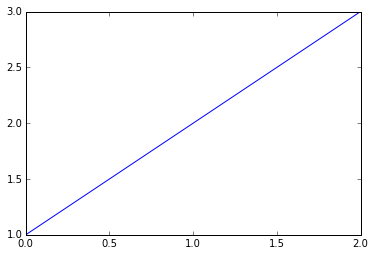### Install Basemap from source¶ These installation instructions are adapted from the Basemap documentation. These may not be the best setup instructions; if you have any feedback, please share it. First you need to download the source code for Basemap, which is a matplotlib toolkit. As of this writing, the newest version of Basemap is 1.0.7. Look for the newest version here, and click the folder. On the next page, look for a file called basemap-1.x.x.tar.gz. This is the file you want to download. It is about 120MB, so again you will need a decent internet connection. When the download is complete, open the archive and drag the basemap-1.x.x folder to your home folder, or /usr/local/, or wherever you keep your source code. You first need to install geos. From the basemap-1.x.x directory, change into the geos directory and set the GEOS_DIR environment variable. These directions assume you are working from your home directory; if you are working in /usr/local/, you will probably need to change the GEOS_DIR to /usr/local/: basemap-1.x.x$ cd geos-3.3.3
basemap-1.x.x/geos-3.3.3 $export GEOS_DIR=~/ basemap-1.x.x/geos-3.3.3$ ./configure --prefix=$GEOS_DIR basemap-1.x.x/geos-3.3.3$ make
basemap-1.x.x/geos-3.3.3 $make install  Now you need to change back to the basemap parent directory, and install basemap: basemap-1.x.x/geos-3.3.3$ cd ..
basemap-1.x.x \$ sudo python setup.py install



You can test if everything worked by running an example. From your basemap-1.x.x directory, change into the examples directory and run simpletest.py. Basemap is a large library, so the example script may take a while to run. You should see something like this:## Making a simple map¶

Let's start out by trying to make a simple map of the world. If you run the following code, you should get a nice map of the globe, with good clean coastlines:

In :
from mpl_toolkits.basemap import Basemap
import matplotlib.pyplot as plt
import numpy as np

# make sure the value of resolution is a lowercase L,
#  for 'low', not a numeral 1
map = Basemap(projection='ortho', lat_0=50, lon_0=-100,
resolution='l', area_thresh=1000.0)

map.drawcoastlines()

plt.show()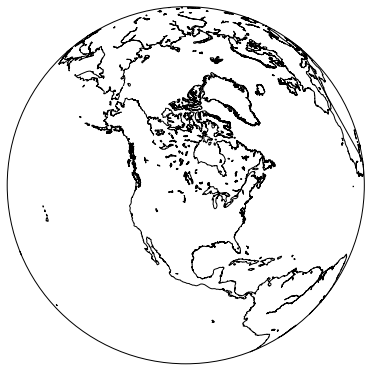If you get a map that looks incomplete, such as the one below, you may want to try installing matplotlib and Basemap from source.Let’s add some more detail to this map, starting with country borders. Add the following lines after map.drawcoastlines():

In :
###highlight=[12,13]
from mpl_toolkits.basemap import Basemap
import matplotlib.pyplot as plt
import numpy as np

# make sure the value of resolution is a lowercase L,
#  for 'low', not a numeral 1
map = Basemap(projection='ortho', lat_0=50, lon_0=-100,
resolution='l', area_thresh=1000.0)

map.drawcoastlines()
map.drawcountries()
map.fillcontinents(color='coral')

plt.show()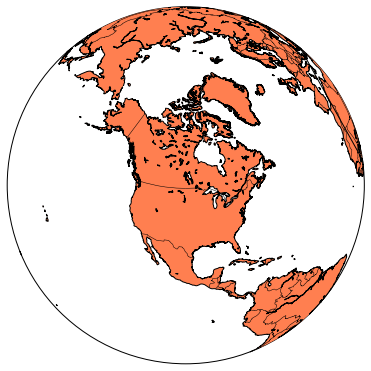You should see the continents filled in. If the entire globe is filled in, you may need to update your version of matplotlib, the basemap toolkit, or both. That said, I watched one student follow this tutorial. The entire globe ended up colored in, but on subsequent runs the colors came out right. I’m pretty sure this is a glitch in either matplotlib or Basemap. If you are having issues with this, you may want to go back and install basemap from source.

Let’s clean up the edge of the globe:

In :
###highlight=
from mpl_toolkits.basemap import Basemap
import matplotlib.pyplot as plt
import numpy as np

# make sure the value of resolution is a lowercase L,
#  for 'low', not a numeral 1
map = Basemap(projection='ortho', lat_0=50, lon_0=-100,
resolution='l', area_thresh=1000.0)

map.drawcoastlines()
map.drawcountries()
map.fillcontinents(color='coral')
map.drawmapboundary()

plt.show()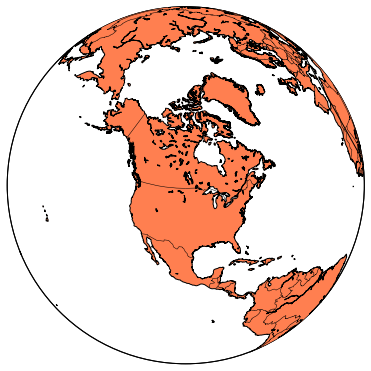You should see a cleaner circle outlining the globe. Now let’s draw latitude and longitude lines:

In :
###highlight=[16,17]
from mpl_toolkits.basemap import Basemap
import matplotlib.pyplot as plt
import numpy as np

# make sure the value of resolution is a lowercase L,
#  for 'low', not a numeral 1
map = Basemap(projection='ortho', lat_0=50, lon_0=-100,
resolution='l', area_thresh=1000.0)

map.drawcoastlines()
map.drawcountries()
map.fillcontinents(color='coral')
map.drawmapboundary()

map.drawmeridians(np.arange(0, 360, 30))
map.drawparallels(np.arange(-90, 90, 30))

plt.show()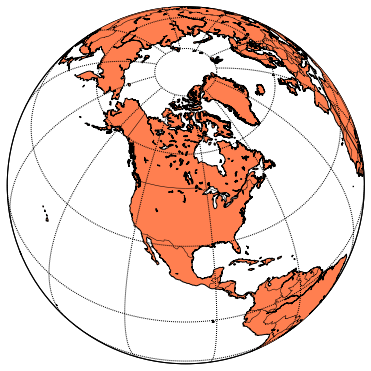The np.arange() arguments tell where your latitude and longitude lines should begin and end, and how far apart they should be spaced.

Let’s play with two of the map settings, and then we will move on to plotting data on this globe. Let’s start by adjusting the perspective. Change the latitude and longitude parameters in the original Basemap definition to 0 and -100. When you run the program, you should see your map centered along the equator:

In :
###highlight=[8,9]
from mpl_toolkits.basemap import Basemap
import matplotlib.pyplot as plt
import numpy as np

# make sure the value of resolution is a lowercase L,
#  for 'low', not a numeral 1
map = Basemap(projection='ortho', lat_0=0, lon_0=-100,
resolution='l', area_thresh=1000.0)

map.drawcoastlines()
map.drawcountries()
map.fillcontinents(color='coral')
map.drawmapboundary()

map.drawmeridians(np.arange(0, 360, 30))
map.drawparallels(np.arange(-90, 90, 30))

plt.show()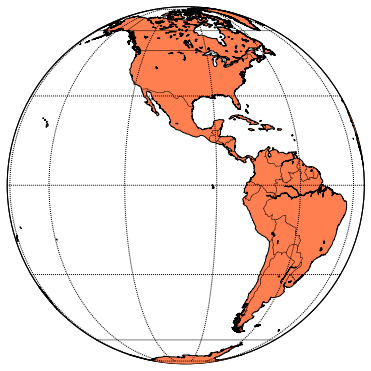Now let’s change the kind of map we are producing. Change the projection type to ‘robin’. You should end up with a Robinson projection instead of a globe:

In :
###highlight=
from mpl_toolkits.basemap import Basemap
import matplotlib.pyplot as plt
import numpy as np

# make sure the value of resolution is a lowercase L,
#  for 'low', not a numeral 1
map = Basemap(projection='robin', lat_0=0, lon_0=-100,
resolution='l', area_thresh=1000.0)

map.drawcoastlines()
map.drawcountries()
map.fillcontinents(color='coral')
map.drawmapboundary()

map.drawmeridians(np.arange(0, 360, 30))
map.drawparallels(np.arange(-90, 90, 30))

plt.show()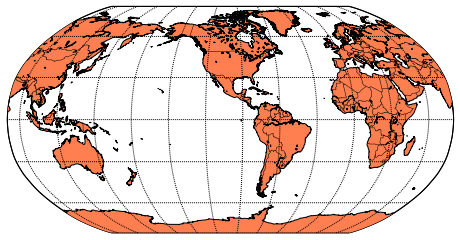## Zooming in¶

Before we move on to plotting points on the map, let’s see how to zoom in on a region. This is good to know because there are many data sets specific to one region of the world, which would get lost when plotted on a map of the whole world. Some projections can not be zoomed in at all, so if things are not working well, make sure to look at the documentation.

I live on Baranof Island in southeast Alaska, so let’s zoom in on that region. One way to zoom in is to specify the latitude and longitude of the lower left and upper right corners of the region you want to show. Let’s use a mercator projection, which supports this method of zooming. The notation for “lower left corner at 136.25 degrees west and 56 degrees north” is:

llcrnrlon = -136.25, llcrnrlat = 56.0



So, the full set of parameters we will try is:

In :
###highlight=[10,11]
from mpl_toolkits.basemap import Basemap
import matplotlib.pyplot as plt
import numpy as np

# make sure the value of resolution is a lowercase L,
#  for 'low', not a numeral 1
map = Basemap(projection='merc', lat_0=57, lon_0=-135,
resolution = 'l', area_thresh = 1000.0,
llcrnrlon=-136.25, llcrnrlat=56,
urcrnrlon=-134.25, urcrnrlat=57.75)

map.drawcoastlines()
map.drawcountries()
map.fillcontinents(color='coral')
map.drawmapboundary()

map.drawmeridians(np.arange(0, 360, 30))
map.drawparallels(np.arange(-90, 90, 30))

plt.show()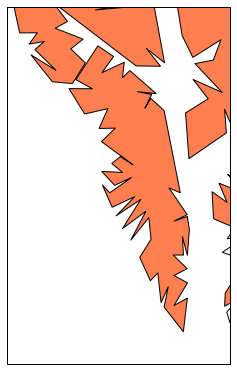Note that the center of the map, given by lat_0 and lon_0, must be within the region you are zoomed in on.

This worked, but the map is pretty ugly. We are missing an entire island to the west of here! Let’s change the resolution to ‘h’ for ‘high’, and see what we get:

In :
###highlight=
from mpl_toolkits.basemap import Basemap
import matplotlib.pyplot as plt
import numpy as np

# make sure the value of resolution is a lowercase L,
#  for 'low', not a numeral 1
map = Basemap(projection='merc', lat_0=57, lon_0=-135,
resolution = 'h', area_thresh = 1000.0,
llcrnrlon=-136.25, llcrnrlat=56,
urcrnrlon=-134.25, urcrnrlat=57.75)

map.drawcoastlines()
map.drawcountries()
map.fillcontinents(color='coral')
map.drawmapboundary()

map.drawmeridians(np.arange(0, 360, 30))
map.drawparallels(np.arange(-90, 90, 30))

plt.show()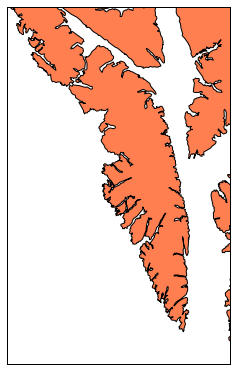This is much better, but we are still missing an entire island to the west. This is because of the area_thresh setting. This setting specifies how large a feature must be in order to appear on the map. The current setting will only show features larger than 1000 square kilometers. This is a reasonable setting for a low-resolution map of the world, but it is a really bad choice for a small-scale map. Let’s change that setting to 0.1, and see how much detail we get:

In :
###highlight=
from mpl_toolkits.basemap import Basemap
import matplotlib.pyplot as plt
import numpy as np

# make sure the value of resolution is a lowercase L,
#  for 'low', not a numeral 1
map = Basemap(projection='merc', lat_0=57, lon_0=-135,
resolution = 'h', area_thresh = 0.1,
llcrnrlon=-136.25, llcrnrlat=56,
urcrnrlon=-134.25, urcrnrlat=57.75)

map.drawcoastlines()
map.drawcountries()
map.fillcontinents(color='coral')
map.drawmapboundary()

map.drawmeridians(np.arange(0, 360, 30))
map.drawparallels(np.arange(-90, 90, 30))

plt.show()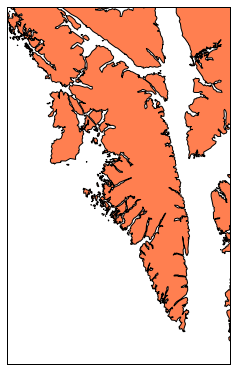This is now a meaningful map. We can see Kruzof island, the large island to the west of Baranof, and many other islands in the area. Settings lower than area_thresh=0.1 do not add any new details at this level of zoom.

Basemap is an incredibly flexible package. If you are curious to play around with other settings, take a look at the Basemap documentation. Next, we will learn how to plot points on our maps.

# Plotting points on a simple map¶

It is a testament to the hard work of many other people that we can create a map like the one above in less than 15 lines of code! Now let’s add some points to this map. I live in Sitka, the largest community on Baranof Island, so let’s add a point showing Sitka’s location. Add the following lines just before plt.show():

In :
###highlight=[16,17,18,19]
from mpl_toolkits.basemap import Basemap
import matplotlib.pyplot as plt
import numpy as np

map = Basemap(projection='merc', lat_0 = 57, lon_0 = -135,
resolution = 'h', area_thresh = 0.1,
llcrnrlon=-136.25, llcrnrlat=56.0,
urcrnrlon=-134.25, urcrnrlat=57.75)

map.drawcoastlines()
map.drawcountries()
map.fillcontinents(color = 'coral')
map.drawmapboundary()

lon = -135.3318
lat = 57.0799
x,y = map(lon, lat)
map.plot(x, y, 'bo', markersize=12)

plt.show()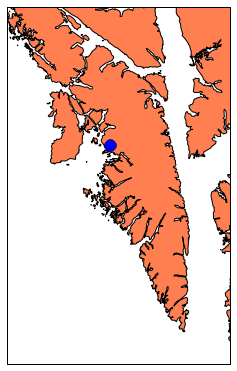The only non-obvious line here is the bo argument, which tells basemap to use a blue circle for the point. There are quite a number of colors and symbols you can use. For more choices, see the documentation for the matplotlib.pyplot.plot function. The default marker size is 6, but that was too small on this particular map. A markersize of 12 shows up nicely on this map.

Plotting a single point is nice, but we often want to plot a large set of points on a map. There are two other communities on Baranof Island, so let’s show where those two communities are on this map. We store the latitudes and longitudes of our points in two separate lists, map those to x and y coordinates, and plot those points on the map. With more dots on the map, we also want to reduce the marker size slightly:

In :
###highlight=
from mpl_toolkits.basemap import Basemap
import matplotlib.pyplot as plt
import numpy as np

map = Basemap(projection='merc', lat_0 = 57, lon_0 = -135,
resolution = 'h', area_thresh = 0.1,
llcrnrlon=-136.25, llcrnrlat=56.0,
urcrnrlon=-134.25, urcrnrlat=57.75)

map.drawcoastlines()
map.drawcountries()
map.fillcontinents(color = 'coral')
map.drawmapboundary()

lons = [-135.3318, -134.8331, -134.6572]
lats = [57.0799, 57.0894, 56.2399]
x,y = map(lons, lats)
map.plot(x, y, 'bo', markersize=10)

plt.show()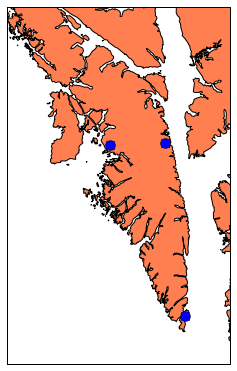## Labeling points¶

Now let’s label these three points. We make a list of our labels, and loop through that list. We need to include the x and y values for each point in this loop, so Basemap can figure out where to place each label.

In :
###highlight=[21,22,23]
from mpl_toolkits.basemap import Basemap
import matplotlib.pyplot as plt
import numpy as np

map = Basemap(projection='merc', lat_0 = 57, lon_0 = -135,
resolution = 'h', area_thresh = 0.1,
llcrnrlon=-136.25, llcrnrlat=56.0,
urcrnrlon=-134.25, urcrnrlat=57.75)

map.drawcoastlines()
map.drawcountries()
map.fillcontinents(color = 'coral')
map.drawmapboundary()

lons = [-135.3318, -134.8331, -134.6572]
lats = [57.0799, 57.0894, 56.2399]
x,y = map(lons, lats)
map.plot(x, y, 'bo', markersize=10)

labels = ['Sitka', 'Baranof Warm Springs', 'Port Alexander']
for label, xpt, ypt in zip(labels, x, y):
plt.text(xpt, ypt, label)

plt.show()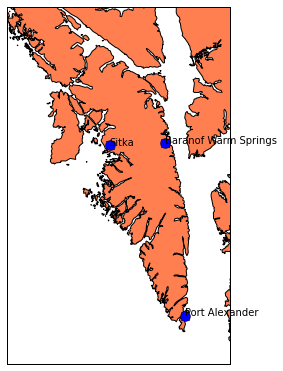Our towns are now labeled, but the labels start right on top of our points. We can add offsets to these points, so they are not right on top of the points. Let’s move all of the labels a little up and to the right. (If you are curious, these offsets are in map projection coordinates, which are measured in meters. This means our code actually places the labels 10 km to the east and 5 km to the north of the actual townsites.)

In :
###highlight=
from mpl_toolkits.basemap import Basemap
import matplotlib.pyplot as plt
import numpy as np

map = Basemap(projection='merc', lat_0 = 57, lon_0 = -135,
resolution = 'h', area_thresh = 0.1,
llcrnrlon=-136.25, llcrnrlat=56.0,
urcrnrlon=-134.25, urcrnrlat=57.75)

map.drawcoastlines()
map.drawcountries()
map.fillcontinents(color = 'coral')
map.drawmapboundary()

lons = [-135.3318, -134.8331, -134.6572]
lats = [57.0799, 57.0894, 56.2399]
x,y = map(lons, lats)
map.plot(x, y, 'bo', markersize=10)

labels = ['Sitka', 'Baranof Warm Springs', 'Port Alexander']
for label, xpt, ypt in zip(labels, x, y):
plt.text(xpt+10000, ypt+5000, label)

plt.show()